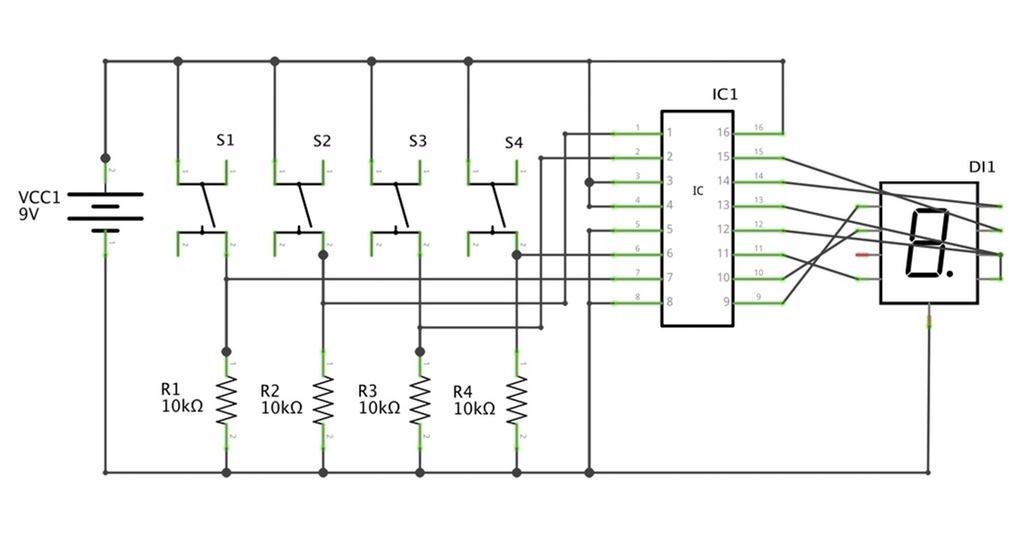## 1s & 0sWhat is binary?

Binary is a way to count numbers (1,2,3,4,5…) in 1 & 0s. A bit weird isn't it? Why would we even bother to count it like that? Well, it's all to do with what we can do with our electronic components.

For example, an LED, it can either be ON or OFF. A transistor, can be either ON or OFF. If we can represent on as 1, and off as 0, then in theory (with binary) we can use them to count! Still a bit hard to understand?

Well, let's push history aside and get down to the counting:

Our numbers (1,2,3,4,5) are called decimal numbers. How many decimal numbers are used to represent a whole number, is called digits.

The decimal number 1 is of one digit.

The decimal number 12 is of two digits.

In binary, digits are called bits.

1 is 1 bit.

11 is 2 bits (1 and 1).

8 bits is a byte. We know all about kilobytes, megabytes and gigabytes, right?